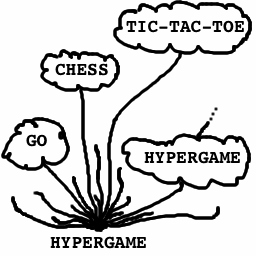# Hypergame## Playing Hypergame

A finite game is a two-player game which is guaranteed to end after a finite number of moves. It is ok for a finite game to have an infinite number of possibilities; for example, the game where player one names any number and immediately wins is a finite game. It is also ok for a game to have an unbounded number of moves, as long as it always ends after finitely many. For example, the game where player one names any number N, then N moves pass with nothing happening before player one wins. This game is still a finite game, since player one will eventually win in a finite number of moves no matter which N was chosen.

To play hypergame, player one names any finite game. Player two then makes the first move in that game, and play continues as usual. Whoever wins the game also wins the hypergame.

## Representing Hypergame

• What does the game tree of hypergame look like?
• Does either player have a winning strategy?
• Is hypergame a finite game?

Since hypergame appears to be a finite game, saying "let's play hypergame" is a legal first move. But then player two must choose a finite game -- for example, hypergame! Now player one gets to choose a finite game, maybe... hypergame! So is hypergame a finite game or not?

This is closely related to a classical paradox of set theory called Russell's Paradox. Russell's paradox goes as follows: out of all the sets that could exist, some sets contain themselves and some do not. Let R be the collection of all sets which do not contain themselves. Now ask yourself: does R contain itself?

R only consists of sets which do not contain themselves. So if R contains itself, then R must be a set which does not contain itself! This is of course a contradiction.

How about the other way around? If R does not contain itself, then by the definition of R it must contain itself! So which is it? Either alternative is contradictory.

There is a similar problem with hypergame: if hypergame is a finite game, then it is a legal first move, in which case hypergame is not a finite game. On the other hand, if hypergame is not a finite game then there is a first move which leads to a non-finite game, but all first moves only lead to finite games.